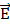# A particle of mass m and charge q is in an electric and magnetic field given by= 2+ 3;= 4+6. The charged particle is shifted from the origin to the point P(x = 1; y = 1) along a straight path. The magnitude of the total work done is : a. (0.35)q b. (0.15)q c. (2.5)q d. 5q

## Question ID - 50613 :- A particle of mass m and charge q is in an electric and magnetic field given by= 2+ 3;= 4+6. The charged particle is shifted from the origin to the point P(x = 1; y = 1) along a straight path. The magnitude of the total work done is : a. (0.35)q b. (0.15)q c. (2.5)q d. 5q

3537= q+q(×)           = (2q+3q) + q(×) W =.= 2q + 3q        =  5q

Next Question :

Two light identical springs of spring constant k are attached horizontally at the two ends of a uniform horizontal rod AB of length l and mass m. The rod is pivoted at its centre 'O' and can rotate freely in horizontal plane. The other ends of the two springs are fixed to rigid supports as shown in figure. The rod is gently pushed through a small angle and released. The frequency of resulting oscillation is:a.b.c.d.